## The glicko rating system

As may have noticed, each FICS player has a rating and an RD.

RD stands for "ratings deviation".

Why a new system?

The new system with the RD improves upon the binary categorization that was used before on fics and elsewhere, where players with fewer than 20 games were labeled "provisional" and others were labeled "established". Instead of two separate ratings formulas for the two categories, there is now a single formula incorporating the two ratings and the two RD's to find the ratings changes for you and your opponent after a game.

What RD represents

The Ratings Deviation is used to measure how much a player's current rating should be trusted. A high RD indicates that the player may not be competing frequently or that the player has not played very many games yet at the current rating level. A low RD indicates that the player's rating is fairly well established. This is described in more detail below under "RD Interpretation".

How RD Affects Ratings Changes

In general, if your RD is high, then your rating will change a lot each time you play. As it gets smaller, the ratings change per game will go down. However, your opponent's RD will have the opposite effect, to a smaller extent: if his RD is high, then your ratings change will be somewhat smaller than it would be otherwise.

A further use of RD's

Vek asked Mark Glickman the following:

```> Given player one with rating r1, error s1,
> and player two with r2 and s2, do you have a formula for the
> probability that player 1's "true" rating is greater than
> player 2's ?```

Mark said:

```  Yes - it's:
1/(1 + 10^(-(r1-r2)f(sqrt(s1^2 + s2^2))/400) )
where f(s) is [the function applied to RD in Step 2 below].```

How RD is Updated

In this system, the RD will decrease somewhat each time you play a game, because when you play more games there is a stronger basis for concluding what your rating should be. However, if you go for a long time without playing any games, your RD will increase to reflect the increased uncertainty in your rating due to the passage of time. Also, your RD will decrease more if your opponent's rating is similar to yours, and decrease less your opponent's rating is much different.

Why Ratings Changes Aren't Balanced

In the other system, except for provisional games, the ratings changes for the two players in a game would balance each other out - if A wins 16 points, B loses 16 points. That is not the case with this system. Here is the explanation I received from Mark Glickman:

The system does not conserve rating points - and with good reason! Suppose two players both have ratings of 1700, except one has not played in awhile and the other playing constantly. In the former case, the player's rating is not a reliable measure while in the latter case the rating is a fairly reliable measure. Let's say the player with the uncertain rating defeats the player with the precisely measured rating. Then I would claim that the player with the imprecisely measured rating should have his rating increase a fair amount (because we have learned something informative from defeating a player with a precisely measured ability) and the player with the precise rating should have his rating decrease by a very small amount (because losing to a player with an imprecise rating contains little information). That's the intuitive gist of my extension to the Elo system.

On average, the system will stay roughly constant (by the law of large numbers). In other words, the above scenario in the long run should occur just as often with the imprecisely rated player losing.

Mathematical Interpretation of RD

Direct from Mark Glickman:

Each player can be characterized as having a true (but unknown) rating that may be thought of as the player's average ability. We never get to know that value, partly because we only observe a finite number of games, but also because that true rating changes over time as a player's ability changes. But we can estimate the unknown rating. Rather than restrict oneself to a single estimate of the true rating, we can describe our estimate as an interval of plausible values. The interval is wider if we are less sure about the player's unknown true rating, and the interval is narrower if we are more sure about the unknown rating. The RD quantifies the uncertainty in terms of probability:

The interval formed by Current rating +/- RD contains your true rating with probability of about 0.67.

The interval formed by Current rating +/- 2 RD contains your true rating with probability of about 0.95.

The interval formed by Current rating +/- 3 RD contains your true rating with probability of about 0.997.

For those of you who know something about statistics, these are not confidence intervals, but are called "central posterior intervals" because the derivation came from a "Bayesian" analysis of the problem.

These numbers are found from the cumulative distribution function of the normal distribution with mean = current rating, and standard deviation = RD. For example, CDF[ N[1600,50], 1550 ] = .159 approximately (that's shorthand Mathematica notation.)

The Formulas

Algorithm to calculate ratings change for a game against a given opponent:

Step 1. Before a game, calculate initial rating and RD for each player.

a) If no games yet, initial rating assumed to be 1720. Otherwise, use existing rating. (The 1720 is not printed out, however.)

b) If no RD yet, initial RD assumed to be 350 if you have no games, or 70 if your rating is carried over from ICC. Otherwise, calculate new RD, based on the RD that was obtained after the most recent game played, and on the amount of time (t) that has passed since that game, as follows: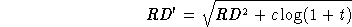where c is a numerical constant chosen so that predictions made according to the ratings from this system will be approximately optimal.

Step 2. Calculate the "attenuating factor" due to your OPPONENT's RD, for use in later steps.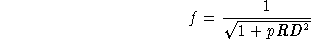Here p is the mathematical constant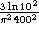.

Note that this is between 0 and 1 - if RD is very big, then f will be closer to 0.

Step 3. r1: your rating, r2: opponent's rating,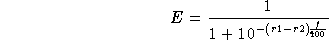(it has f(RD) in it!)

This quantity E seems to be treated kind of like a probability.

Step 4.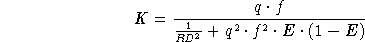where q is a mathematical constant: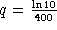.

Step 5. This is the K factor for the game, so

Your new rating = (pregame rating) + K(w - E)

where w is 1 for a win, .5 for a draw, and 0 for a loss.

Step 6. Your new RD is calculated as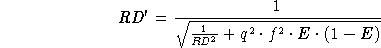The same steps are done for your opponent.

Credits

The Glicko Ratings System was invented by Mark Glickman, Ph.D. who is currently at the Harvard Statistics Department, and who is bound for Boston University.

Vek and Hawk programmed and debugged the new ratings calculations (we may still be debugging it). Helpful assistance was given by Surf, and Shane fixed a heinous bug that Vek invented.

Vek wrote this helpfile and Mark Glickman made some essential corrections and additions. Last updated April 19, 1995.